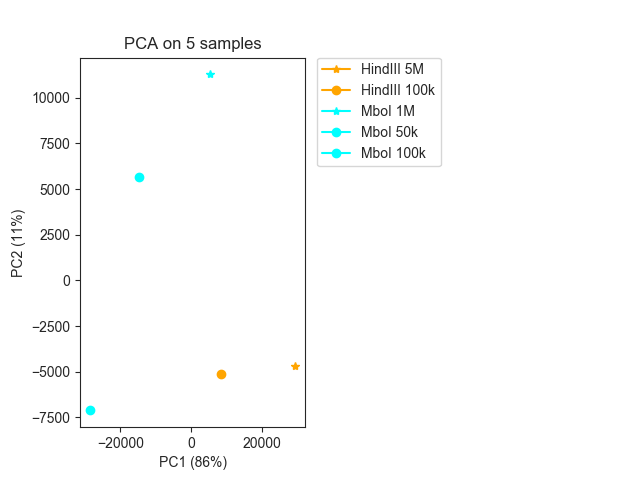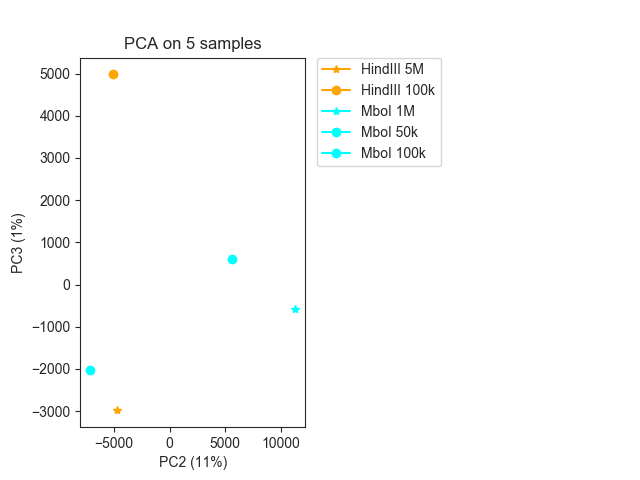# PCA¶

```import fanc
import fanc.plotting as fancplot
from fanc.architecture.comparisons import hic_pca

```

PCA is one way in FAN-C to compare different Hi-C matrices to each other. A matrix of pixels vs matrices is assembled that contains the (normalised) contact strength of each matrix for the respective pixel (=region pair). PCA is then run on this matrix, and the resulting eigenvectors can be plotted to examine the variability between datasets.

In FAN-C, simply use `hic_pca()` for this purpose, as shown here for chromosome 19:

```pca_info, pca_result = hic_pca(lowc_hindiii_5M, lowc_hindiii_100k,
lowc_mboi_1M, lowc_mboi_50k,
lowc_mboi_100k, ignore_zeros=True,
scale=False, region='chr19', sample_size=100000,
strategy='variance')
```

We can plot the result using `pca_plot()`:

```fig, ax = fancplot.pca_plot(pca_result, variance=pca_info,
names=["HindIII 5M", "HindIII 100k",
"MboI 1M", "MboI 50k", "MboI 100k"])
```We can easily change the colors and markers, for example by colouring all samples with MboI and HindIII differently, and assigning different markers to samples with more or less than 1M cells:

```fig, ax = fancplot.pca_plot(pca_result, variance=pca_info,
names=["HindIII 5M", "HindIII 100k",
"MboI 1M", "MboI 50k", "MboI 100k"],
colors=["orange", "orange", "cyan", "cyan", "cyan"],
markers=["*", "o", "*", "o", "o"])
```Sometimes the first eigenvector captures the library sequencing depth, so you may want to plot the second and third EVs instead using `eigenvectors=(1,2)` (in this case it does not seem to be particularly informative):

```fig, ax = fancplot.pca_plot(pca_result, variance=pca_info,
eigenvectors=(1, 2),
names=["HindIII 5M", "HindIII 100k",
"MboI 1M", "MboI 50k", "MboI 100k"],
colors=["orange", "orange", "cyan", "cyan", "cyan"],
markers=["*", "o", "*", "o", "o"])
```This kind of analysis can be tricky, and selecting informative pixels from the matrix can be key to getting a robust and intuitive PCA result. In the above example, we are using several parameters to select informative pixels for the PCA. First, we are only using pixels that are non-zero in all samples with `ignore_zeros=True`. Second, we are sorting the pixels, listing the ones with the largest variance across samples first, using `strategy='variance'`. Finally, we are selecting the top 100k pixels (those with the largest variance) first with `sample_size=100000`.

When you are analysing matrices of higher resolution, pixels far away from the diagonal might be dominated by noise. `ignore_zeros` removes most of the noisy pixels, but additionally you might want to set a `max_distance` to only select pixels corresponding to regions closer than this value. Similarly, if you want to exclude contacts close to the diagonal, use `min_distance`.

For more options have a look at the API reference for `pca_plot()`.